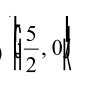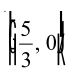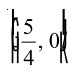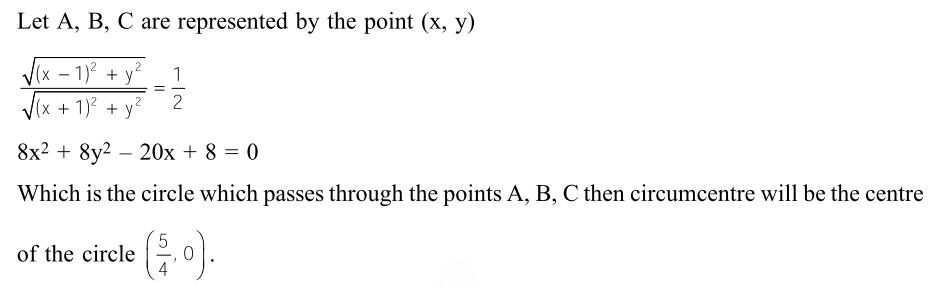# Three distinct points A, B and C are given in the 2–dimensional`
Question:

Three distinct points $\mathrm{A}, \mathrm{B}$ and $\mathrm{C}$ are given in the 2 -dimensional coordinate plane such that the ratio of the distance of any one of them from the point $(1,0)$ to the distance from the point $(-1,0)$ is equal to $\frac{1}{3}$. Then the circumcentre of the triangle $\mathrm{ABC}$ is at the point :-

1.2.3. $(0,0)$

4.Correct Option: , 4

Solution: